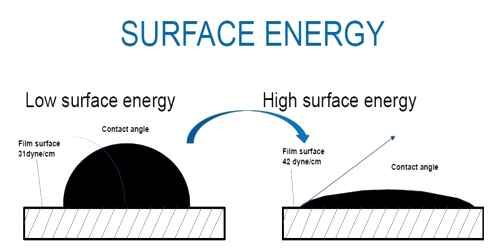# Surface Energy

Surface Energy

We know, a tension or force always exists on the surface of a liquid and this tension tends to decrease the area of the surface of the liquid. So, to increase the area of the surface of a liquid, work must be done against the tension. This work remains stored as potential energy on the surface. This potential energy is apparently called surface energy. So, surface energy is defined as follows:

The amount of work done to increase the surface area by unity is called surface energy. It is generally denoted by E.

So, Surface energy is the work per unit area done by the force that creates the new surface. The molecules on the surface of a liquid are packed due to unbalanced inter-molecular forces than the molecules at the center. This means there is a high energy density at the surface of a liquid.

Unit of surface energy: The S.I. unit of surface energy is Joule/metre2 = (Jm-2).

But, J/m2 = Nm/m2 = Nm-1

So, the unit of surface energy and surface tension is identical.

Dimension of surface energy:

Dimension of surface energy = Dimension of work/Dimension of area

= [Force x Displacement] / [Area]

= [(MLT-2 x L) / L2] = [MT-2]

If amount of work done is W to increase the area of an open surface by ΔA, then surface energy- E = W / ΔA